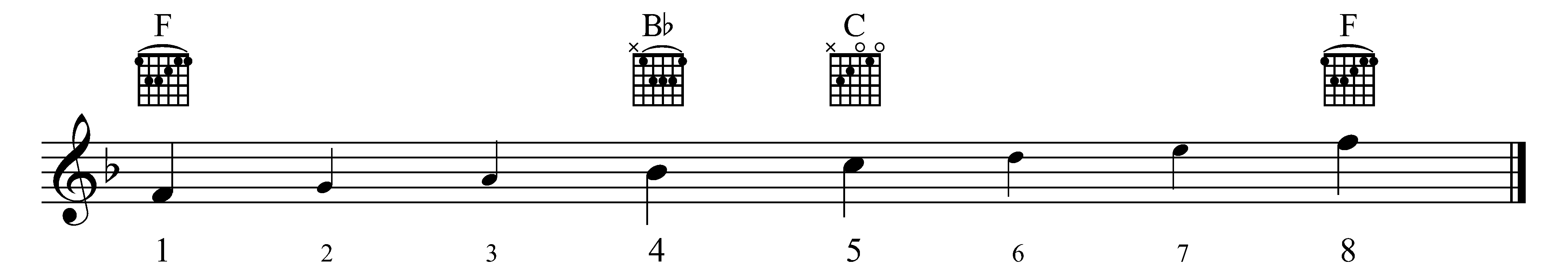# A3 | Chords – root position

Look at the scale below – F major. In A2: primary triads, you saw that the three following chords are the most important in a key a that you can write them using Roman numerals, i.e.:

I = tonic chord (F) IV = subdominant chord (B) V = dominant chord (C)

In figure 1, each chord is in root position, so:

• The chord of the tonic is based on the tonic note. Therefore, the note F is the root of the chord.
• The subdominant chord is based on the subdominant note. Therefore, the note B is the root of the chord.
• The dominant chord is based on the dominant note. Therefore, the note C is the root of the chord.

## F major scale

Fig. 1Tonic (I)

subdominant (IV)

dominant (V)

tonic (I)

Glan Rondda

Dawel Nos